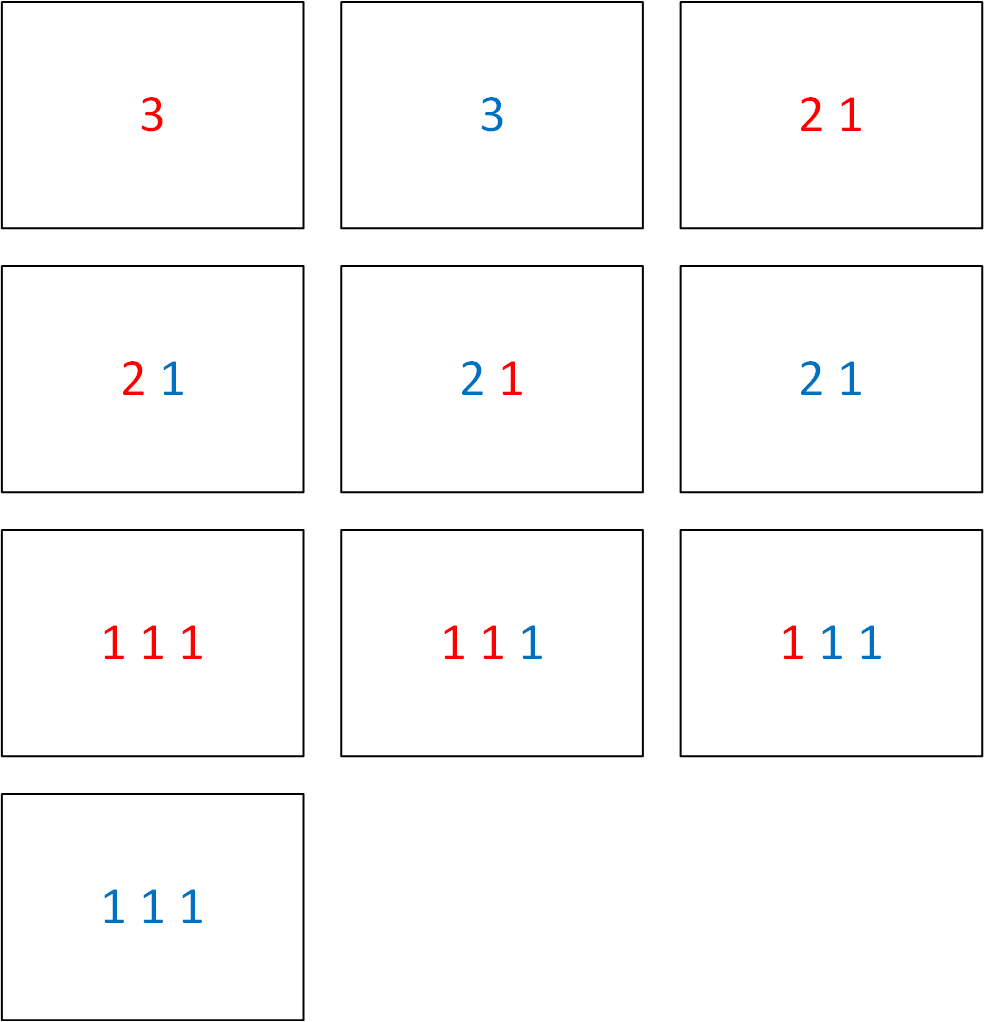시간 제한메모리 제한제출정답맞힌 사람정답 비율
1 초 512 MB17101058.824%

## 문제

Kate's school has introduced multiple subject lessons.

The students have the following task for the lesson of Math, Art and Sociology in the seventh grade. They are given an integer $n$. Each student has a set of $k$ colored pencils, let the colors be numbered from $1$ to $k$. Each students takes a sheet of paper and writes down one or several integers at it, so that their sum was equal to $n$. Each integer is written using one of the pencils, so it has one of the $k$ possible colors.

The students must agree to do the task in such way that no two students have the same solution. Two solutions are the same if for each integer $a$ and each color $i$ the number of integers $a$ of color $i$ at students' sheets is the same.

The teacher of Math is sure that the students will be able to complete the task. However, she wants to know how many solutions are there, maybe there are not enough for all the students to have different solutions. Help her to find that out!

## 입력

The input contains two integers $n$ and $k$ ($1 \le n, k \le 15$).

## 출력

Print one integer --- the number of solutions to the task.

## 예제 입력 1

3 2


## 예제 출력 1

10


## 힌트

The following picture shows all possible ways to solve the task in the first sample test. Note that the order of integers written doesn't matter, only the number of integers written with each color.